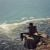# János Komlós (mathematician)

Updated on
Covid-19
 Name  Janos KomlosRole  Mathematician Awards  Alfred Renyi Prize Education  Eotvos Lorand University

János Komlós (Budapest, 23 May 1942) is a Hungarian-American mathematician, working in probability theory and discrete mathematics. He has been a professor of mathematics at Rutgers University since 1988. He graduated from the Eötvös Loránd University, then became a fellow at the Mathematical Institute of the Hungarian Academy of Sciences. Between 1984–1988 he worked at the University of California, San Diego.

## Notable results

• He proved that every L1-bounded sequence of real functions contains a subsequence such that the arithmetic means of all its subsequences converge pointwise almost everywhere. In probabilistic terminology, the theorem is as follows. Let ξ12,... be a sequence of random variables such that E1],E2],... is bounded. Then there exist a subsequence ξ'1, ξ'2,... and a random variable β such that for each further subsequence η12,... of ξ'0, ξ'1,... we have (η1+...+ηn)/n → β a.s.
• With Ajtai and Szemerédi he proved the ct2/log t upper bound for the Ramsey number R(3,t). The corresponding lower bound was proved by Kim only in 1995, this result earned him a Fulkerson Prize.
• The same team of authors developed the optimal Ajtai–Komlós–Szemerédi sorting network.
• Komlós and Szemerédi proved that if G is a random graph on n vertices with
• 1 2 n log n + 1 2 n log log n + c n
edges, where c is a fixed real number, then the probability that G has a Hamiltonian circuit converges to
e e 2 c .
• With Gábor Sárközy and Endre Szemerédi he proved the so-called blow-up lemma which claims that the regular pairs in Szemerédi's regularity lemma are similar to complete bipartite graphs when considering the embedding of graphs with bounded degrees.
• Komlós worked on Heilbronn's problem; he, János Pintz and Szemerédi disproved Heilbronn's conjecture.
• Komlós also wrote highly cited papers on sums of random variables, space-efficient representations of sparse sets, random matrices, the Szemerédi regularity lemma, and derandomization.
• ## Degrees, awards

Komlós received his Ph.D. in 1967 from Eötvös Loránd University under the supervision of Alfréd Rényi. In 1975 he received the Alfréd Rényi Prize, a prize established for researchers of the Alfréd Rényi Institute of Mathematics. In 1998 he was elected as an external member to the Hungarian Academy of Sciences.

Topics